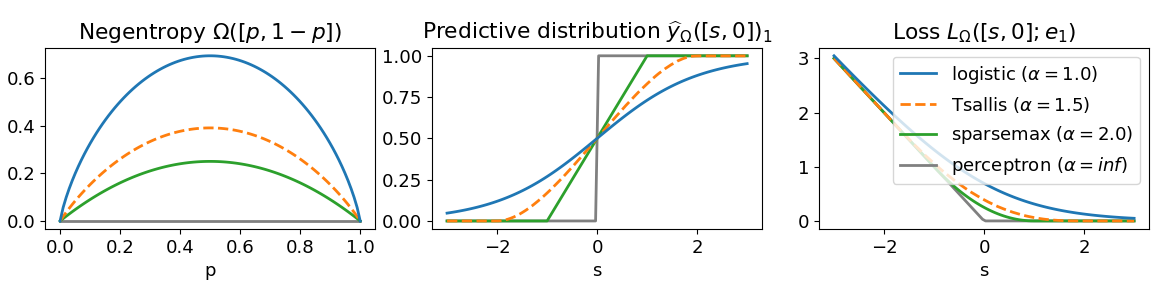# Fenchel Young Losses

Probabilistic classification in PyTorch/TensorFlow/scikit-learn with Fenchel-Young losses
Alternatives To Fenchel Young Losses
Transformers89,2126491115 hours ago91June 21, 2022637apache-2.0Python
🤗 Transformers: State-of-the-art Machine Learning for Pytorch, TensorFlow, and JAX.
Real Time Voice Cloning40,272
11 days ago104otherPython
Clone a voice in 5 seconds to generate arbitrary speech in real-time
Ray24,8538019913 hours ago76June 09, 20222,893apache-2.0Python
Ray is a unified framework for scaling AI and Python applications. Ray consists of a core distributed runtime and a toolkit of libraries (Ray AIR) for accelerating ML workloads.
Netron21,82146317 hours ago489July 04, 202222mitJavaScript
Visualizer for neural network, deep learning, and machine learning models
D2l En17,205
2 days ago87otherPython
Interactive deep learning book with multi-framework code, math, and discussions. Adopted at 400 universities from 60 countries including Stanford, MIT, Harvard, and Cambridge.
Ncnn16,782
14 hours ago19July 01, 2022958otherC++
ncnn is a high-performance neural network inference framework optimized for the mobile platform
Datasets15,6609208a day ago52June 15, 2022533apache-2.0Python
🤗 The largest hub of ready-to-use datasets for ML models with fast, easy-to-use and efficient data manipulation tools
Deeplearning Models15,594
2 months ago5mitJupyter Notebook
A collection of various deep learning architectures, models, and tips
Onnx14,37514824513 hours ago26June 18, 2022381apache-2.0Python
Open standard for machine learning interoperability
Best Of Ml Python13,176
2 days ago15cc-by-sa-4.0
🏆 A ranked list of awesome machine learning Python libraries. Updated weekly.
Alternatives To Fenchel Young Losses
Select To Compare

Alternative Project Comparisons

# Fenchel-Young losses

This package implements loss functions useful for probabilistic classification. More specifically, it provides

• drop-in replacements for PyTorch loss functions
• drop-in replacements for TensorFlow loss functions
• scikit-learn compatible classifiers

The package is based on the Fenchel-Young loss framework [1,2,3].Notice from the center plot that sparsemax and Tsallis are able to produce exactly zero (sparse) probabilities unlike the logistic (softmax) loss.

## Supported Fenchel-Young losses

• Multinomial logistic loss
• One-vs-all logistic loss
• Sparsemax loss (sparse probabilities!)
• Tsallis losses (sparse probabilities!)

Sparse means that some classes have exactly zero probability, i.e., these classes are irrelevant.

Tsallis losses are a family of losses parametrized by a positive real value . They recover the multinomial logistic loss with =1 and the sparsemax loss with =2. Values of between 1 and 2 enable to interpolate between the two losses.

In all losses above, the ground-truth can either be a n_samples 1d-array of label integers (each label should be between 0 and n_classes-1) or a n_samples x n_classes 2d-array of label proportions (each row should sum to 1).

## Examples

scikit-learn compatible classifier:

```import numpy as np
from sklearn.datasets import make_classification
from fyl_sklearn import FYClassifier

X, y = make_classification(n_samples=10, n_features=5, n_informative=3,
n_classes=3, random_state=0)
clf = FYClassifier(loss="sparsemax")
clf.fit(X, y)
print(clf.predict_proba(X[:3]))
```

Drop-in replacement for PyTorch losses:

```import torch
from fyl_pytorch import SparsemaxLoss

# integers between 0 and n_classes-1, shape = n_samples
y_true = torch.tensor([0, 2])
# model scores, shapes = n_samples x n_classes
theta = torch.tensor([[-2.5, 1.2, 0.5],
[2.2, 0.8, -1.5]])
loss = SparsemaxLoss()
# loss value (caution: reversed convention compared to numpy and tensorflow)
print(loss(theta, y_true))
# predictions (probabilities) are stored for convenience
print(loss.y_pred)
# can also recompute them from theta
print(loss.predict(theta))
# label proportions are also allowed
y_true = torch.tensor([[0.8, 0.2, 0],
[0.1, 0.2, 0.7]])
print(loss(theta, y_true))
```

Drop-in replacement for tensorflow losses:

```import tensorflow as tf
from fyl_tensorflow import sparsemax_loss, sparsemax_predict

# integers between 0 and n_classes-1, shape = n_samples
y_true = tf.constant([0, 2])
# model scores, shapes = n_samples x n_classes
theta = tf.constant([[-2.5, 1.2, 0.5],
[2.2, 0.8, -1.5]])
# loss value
print(sparsemax_loss(y_true, theta))
# predictions (probabilities)
print(sparsemax_predict(theta))
# label proportions are also allowed
y_true = tf.constant([[0.8, 0.2, 0],
[0.1, 0.2, 0.7]])
print(sparsemax_loss(y_true, theta))
```

## References

  SparseMAP: Differentiable Sparse Structured Inference. Vlad Niculae, Andr F. T. Martins, Mathieu Blondel, Claire Cardie. In Proc. of ICML 2018. [arXiv]
  Learning Classifiers with Fenchel-Young Losses: Generalized Entropies, Margins, and Algorithms. Mathieu Blondel, Andr F. T. Martins, Vlad Niculae. In Proc. of AISTATS 2019. [arXiv]
  Learning with Fenchel-Young Losses. Mathieu Blondel, Andr F. T. Martins, Vlad Niculae. Preprint. [arXiv]

## Author

• Mathieu Blondel, 2018
Popular Pytorch Projects
Popular Tensorflow Projects
Popular Machine Learning Categories
Related Searches

Get A Weekly Email With Trending Projects For These Categories
No Spam. Unsubscribe easily at any time.
Python
Pytorch
Tensorflow
Probability
Sklearn